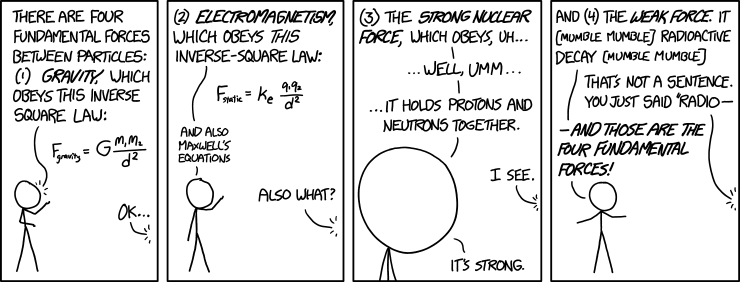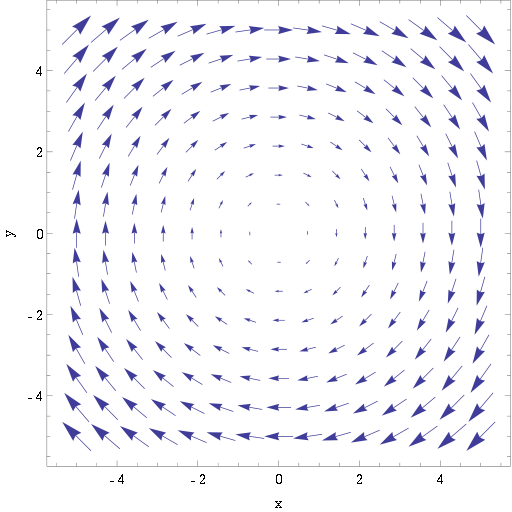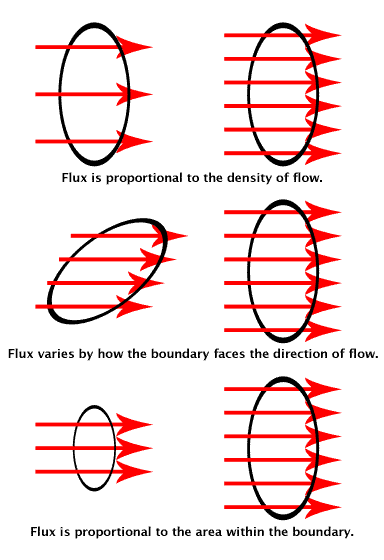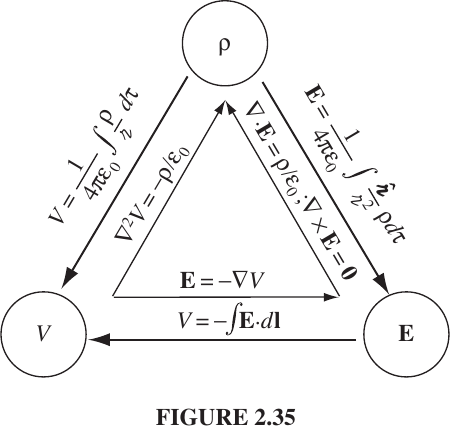# 21

Epistemic Status: The mathematical formulation of electromagnetism is the most solidly established theory in all of science. If this book is wrong then science is broken to the core, space is flat, Colombus' landing in the New World was faked, birds aren't real and the Illuminati controls the lizard people.

There are three fundamental forces. The color force holds atomic nuclei together. (The strong nuclear force is a special case of the color force.) Gravity holds planets and larger structures together. The electroweak force does everything in-between.

The electroweak force unifies the weak nuclear force with the electromagnetic force. The weak nuclear force doesn't matter.When I ask physics majors "What is your favorite textbook?" the answer is usually Introduction to Electrodynamics by David Griffiths. Electrodynamics (in the framework of quantum mechanics) explains basically everything on Earth except meteors and nuclear decay. Electrodynamics is important, satisfying and elegant. It is the basis of almost everything of consequence to human beings.

Griffiths' book is equivalent to at least two months of full-time intermediate-level undergraduate physics at a university.

# Vector Calculus

Like every great physics book, Introduction to Electrodynamics frontloads its math. The math is all vector calculus. If you know vectors and you know calculus then the math should be within your grasp. If you can read and understand the following definition of then you're probably fine.

The most domain-specific math is the use of divergence and curl.

## Divergence

Imagine a vector field as a bunch of arrows. Define a finite region. Divergence is the number of arrows going out of the region minus the arrows going into it.## Curl

Curl is the counterpart of divergence. Imagine again a vector field as a bunch of arrows. Nonzero curl means the arrows go in a circle.# Electostatics

## Coulomb's Law

Electrostatics is governed by Coulomb's Law. The force between two charges is proportional to the inverse square distance between them. Opposite charges attract. Identical charges repel.

The constant is called (ludicrously) the permittivity of free space.

If you think "permittivity of free space" is a silly name then you can call it "vacuum permittivity" or the "electric constant" instead.

## Electric Field

Instead of forces, another equivalent way to model electrostatics is with an electric field which applies a force on charge .

The electric field the same as the Coulomb force except you divide out .

Since we're dealing with vector fields, everything can be superimposed. The electric field of several charges equals the total field at point of each of them put together.

Whether the electric field is "real" is a philosophical question irrelevant to our purposes. What matters is that the electric field formulation of electrostatics is equivalent to the Coulomb force formulation.

## Continuous Charge Distributions

In the previous section we defined electric field of point charges. To get the electric field of a continuous charge distribution you turn the charge into a charge density and integrate over the charge density. You can integrate over one, two or three dimensions for line charges, surface charges and volume charges respectively.

The volumetric formulation is known as Coulomb's law because it is the most general.

## FluxAnother tool for thinking about electric fields is flux. The flux of through a surface is the number of field lines going through it forwards minus the number of field lines going backwards.Electric field lines start at positive charges (or infinity) and terminate at negative charges (or infinity). The flux through a closer surface is therefore a measure of the total charge inside. This is called Gauss's law.

We can generalize the equation to continuous charge distributions by using divergence.

The curl of an electric field is always zero because an electric field cannot point in circles because if it did then you could build a perpetual motion machine by placing an electric charge on a fixed track with nonzero curl.

## Electric Potential

Electric fields are vector fields. It would be better if instead of a three-dimensional value at every point we have a single scaler value instead. We can model an electric field as the gradient of an electric potential because the curl of an electric field is always zero.

The electric potential is the integral of the electric field. The electric potential uniquely determines the electric field. The electric field determines the electric potential up to a constant.

The variable represents a point we have arbitrarily defined to be zero potential. Thus, technically, "potential" has no physical significance. Only differences in potential are physically meaningful. However, it is a good convention to pick to be infinitely far away from your charges. I will use the "zero potential at infinity" convention unless otherwise noted.

The vector components of the electric field look independent but they're actually not because .

Potential obeys the superposition principle. Electric potential has units of joules per coulomb. (Electric fields has units of newtons per coulomb.) The electric field of a charge is proportional to your distance from the charge.

## Poisson's Equation

Recall that the divergence of an electric field equals the charge density.

The electric field is the gradient of the potential. Thus, the divergence of the gradient of the potential equals charge density. The divergence of a gradient is called a Laplacian . Poisson's equation says the Laplacian of an electric potential is proportional to the charge density.

Poisson's equation is useful in quantum field theory.

## Checkpoint

Putting together the equations we have so far, we find that charge density is equivalent to electric field is equivalent (up to an arbitrary constant) to potential .## Epilogue: Work and Energy

Work is the dot product of force and distance.

In the electrostatic context, potential is potential energy per unit charge. The energy of a continuous charge distribution is the charge times the potential.

We can re-write this equation in terms of electric field.

General relativity is beyond the scope of this article but if you've ever wondered where the mass-energy of an electric field comes from here's your answer.

Note that the energy of an electric field does not obey the superposition principle. If you double the electric field you quadruple the energy density.

1. To learn quantum mechanics, check out Introduction to Quantum Mechanics by David Griffiths. ↩︎

# 21

New Comment

I mean, it has been a while since I hung out with the youths. Maybe we only liked Sakurai in my day because we were hipsters.

Typos:

* "The force between two charges  is proportional [to] the inverse square distance  between them."
* "In the previous section we defined [e]lectric field of point charges. To get the electric [field] of a continuous charge distribution you turn the charge  into a charge density  and integrate the electric field [over?] the charge density."
* "Elect[r]ic field lines start at positive charges (or infinity) and terminate at negati[v]e charges (or infinity). The flux through a close[d] surface is therefore a measure of the total charge inside. T[h]is is called Gauss's law."
* "Electric fields are vector fields. It would be better if instead of a three-dimensional value at every point we have a single scal[a]r value instead."
* "The electric potential is the integral of the electric field. The electric potential uniquely determines the electric field. The electric field determines the electric [potential] up to a constant."
* "Note that the energy of an electric field [does] not obey the superposition principle. If you double the electric field you quadruple the energy density."

I put in all the ones I found, but most of them are not consequential. However, several of them could be fairly confusing: "charge" instead of "field", "closer" instead of "closed", "field" instead of "potential".

Thanks. I forgot to spellcheck this post before posting it. I fixed the other spellings that aren't words.

An apparent bug in the LW comment editor prevented me from continuing that comment, simply eating the comment if I typed any more, so I will continue in a reply:

I think overall this post would be hard to follow for someone who didn't already know much of what was in it. This might be less true for people with a stronger calculus background than I have; I mostly have to work out the calculus bits by going backwards from the physics bits.